# Give the thermodynamic criteria for equilibrium(7 MARKS)(At least 2 pages)

Give the thermodynamic criteria for equilibrium(7 MARKS)(At least 2 pages)

~For a system to be in thermodynamic equilibrium, there should be no differences in temperature, pressure, or chemical potential of any of its components. (Chemical potential is the free energy/mole of substance.)

If these conditions are met, the system will not appear to change at the macroscopic level. Nothing will flow. No heat transfers. No material transfers (e.g., evaporation, precipitation, mixing), and no chemical reaction.

A system is said to be in Thermodynamic Equilibrium if it is in phase, thermal, mechanical and chemical equilibrium at same time. If any one of the equilibrium condition disturb then the system cannot comes under Thermodynamic Equilibrium. Let us understand these four equilibrium.

THERMAL EQUILIBRIUM -

The system is said to be in thermal equilibrium if it has equality of temperature with surrounding. For example a dead body is the perfect example of thermal equilibrium with surrounding.

Normal human body temperature is approximately 37 degree Celsius whereas temperature of surrounding keeps on changing, let us consider surrounding temperature is 25 degree Celsius. Therefore a living human body is a not under thermal equilibrium with surrounding because metabolism of the body is continuously working or producing heat. The metabolism of a dead body shuts down therefore after sometime dead body comes under same temperature with surrounding.

PHASE EQUILIBRIUM -

When mass of each phase remains constant with time then the system is in phase Equilibrium. For example -Let us consider a closed container that is partially filled with liquid. Now what happened is evaporation takes place inside the container. The air above the liquid can absorb the water vapour molecules upto a certain limit and this limit is known as saturation limit. This closed container is our system and remember I am considering atmospheric pressure and temperature conditions which means pressure and temperature are not changing. When saturation limit occurs then the mass of liquid present in container will remain constant and similarly mass of vapour present in container will also remain constant. At saturation limit condition there is no more tendency of phase change, so at this point our system is in phase equilibrium.

CHEMICAL EQUILIBRIUM -

Chemical equilibrium is the state when both reactants and products are present in concentrations and have no further tendency to change with time, so that there is no observable change in properties of system. Generally it occurs when rate of forward reaction is equal to rate of backward reaction. In simple language we can say chemical reaction potential should not be present.

Mechanical Equilibrium -

A system is in mechanical equilibrium when net forces acting on that system will be zero. It is basically equality of forces. It can also be defined as, the the pressure acting at any point of the system does not change with time.

In Thermodynamic equilibrium is death. Energy can be extracted from a system until it reaches thermodynamic equilibrium whereupon no more energy can be extracted. A battery that is in thermodynamic equilibrium is a dead battery. If you want to extract energy from a system, that system must be far from equilibrium.

There is an equation that we use to calculate the amount of energy that can be extracted from a system and put to useful work. It is called the Gibbs Equation:

ΔG=ΔG∘+RTlnQΔG=ΔG∘+RTlnQ

The ΔG∘ΔG∘ term fixes the equilibrium position of the reaction. The RTlnQRTlnQ term tells you how far away from equilibrium you are and the ΔGΔG term tells you how much energy can be extracted from the system. If the system is at equilibrium (Q = Keq) then ΔG = 0.

#### Earn Coin

Coins can be redeemed for fabulous gifts.

Similar Homework Help Questions
• ### Page 2 of 17 pages 1 Give brief, clear explanations for the following observations 120 marks tota...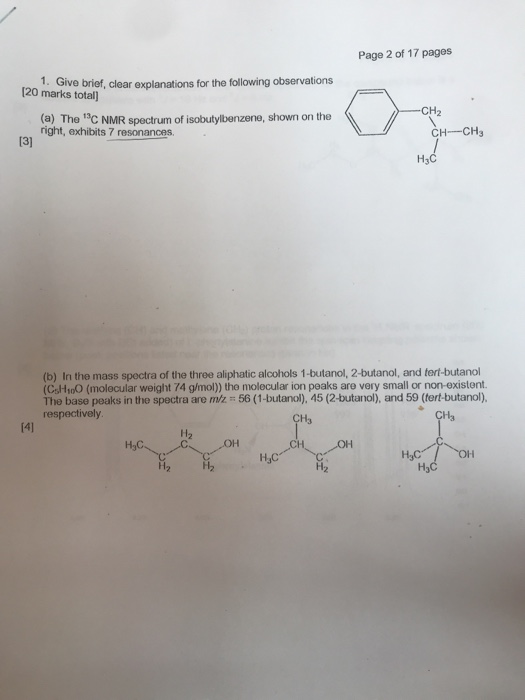Page 2 of 17 pages 1 Give brief, clear explanations for the following observations 120 marks total CH2 (a) The 13c NMR spectrum of isobutylbenzene, shown on the right, exhibits 7 resonances. CH CH 13] H3C ње (b) In the mass spectra of the three aliphatic alcohols 1-butanol, 2-butanol, and tert-butanol (CsH1o0 (molecular weight 74 g/mol) the molecular ion peaks are very small or non-existent. The base peaks in the spectra are miz 56 (1-butanol), 45 (2-butarnol), and 59 (tert-butanol),...

• ### Explain at least three criteria that need to be present for substance dependence according to the...

Explain at least three criteria that need to be present for substance dependence according to the DSM-IV. Explain and give an example of each of the four criteria used to define drug abuse according to the DSM-IV.

• ### 7. Write the at least three differences between kinetic enolate and thermodynamic enolate. 8. Write all...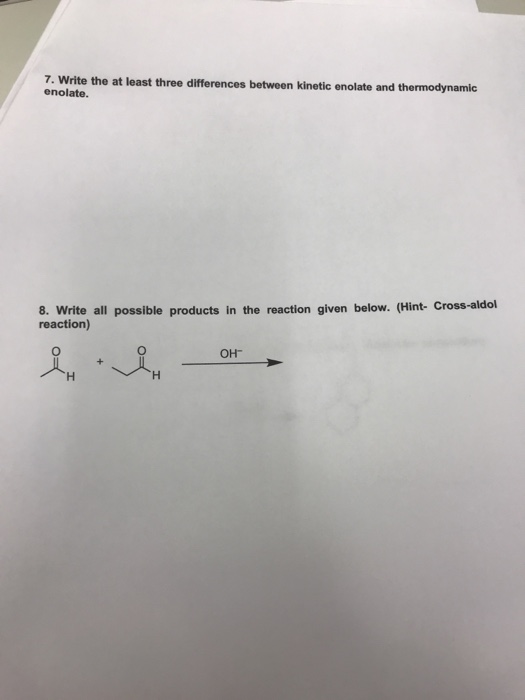7. Write the at least three differences between kinetic enolate and thermodynamic enolate. 8. Write all possible products in the reaction given below. (Hint- Cross-aldol reaction) он-

• ### (Rosen 9.6.52) Give an example of an infinite lattice that fulfills the following criteria. Justi...

(Rosen 9.6.52) Give an example of an infinite lattice that fulfills the following criteria. Justify your answer. (a) neither a least nor a greatest element. (b) a least but not a greatest element. (c) a greatest but not a least element. (d) both a least and a greatest element.

• ### please give a structure that follows the following criteria. Compound C Molecular Formula C12H22 1. 2....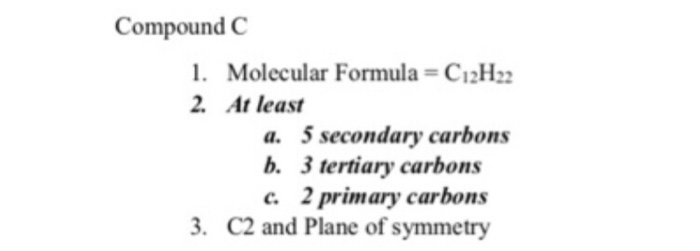please give a structure that follows the following criteria. Compound C Molecular Formula C12H22 1. 2. At least a. 5 secondary carbons b. 3 tertiary carbons . 2 primary carbons 3. C2 and Plane of symmetry Compound C Molecular Formula C12H22 1. 2. At least a. 5 secondary carbons b. 3 tertiary carbons . 2 primary carbons 3. C2 and Plane of symmetry

• ### Use the data below, for 298.15 K, to calculate the thermodynamic equilibrium constant, kp, at 839...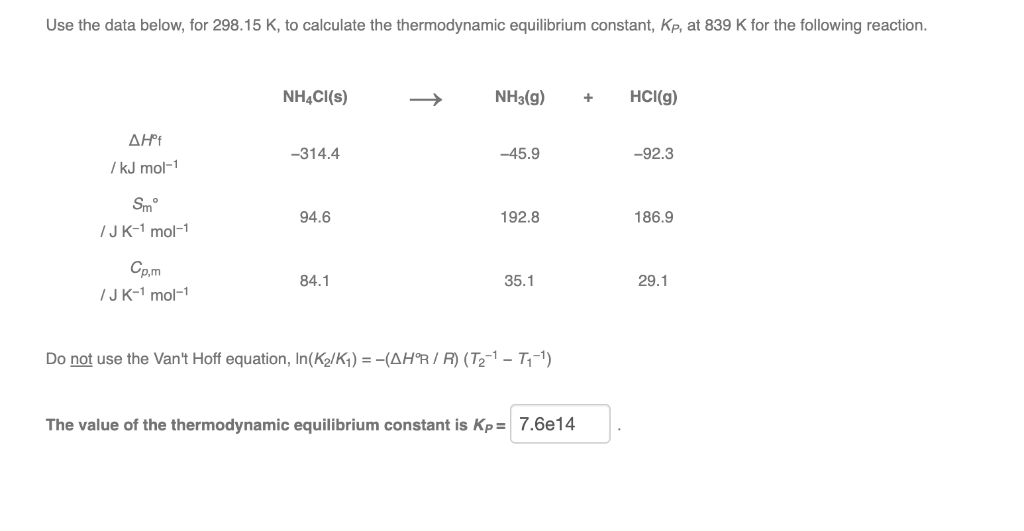Use the data below, for 298.15 K, to calculate the thermodynamic equilibrium constant, kp, at 839 K for the following reaction. NH4Cl(s) NH3(g) + HCl(g) ΔΗ 7 kJ mol-1 -314.4 -45.9 -92.3 Smº JK-1 mol-1 94.6 192.8 186.9 Cp,m 84.1 35.1 29.1 /JK-1 mol-1 Do not use the Van't Hoff equation, In(K /K1) = -(AHR/R) (T2-1 - 7,-1) The value of the thermodynamic equilibrium constant is Kp= 7.6e14

• ### Given the thermodynamic data below, calculate the value of the equilibrium constant for the reaction shown...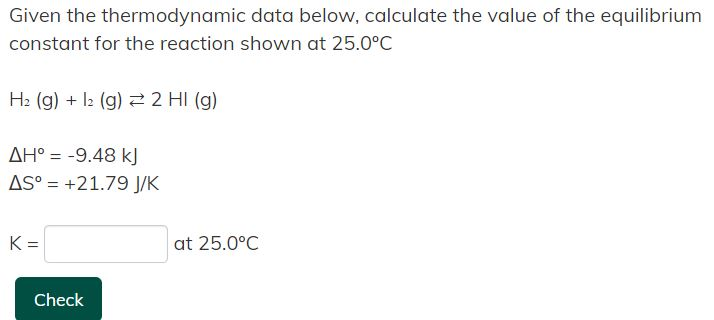Given the thermodynamic data below, calculate the value of the equilibrium constant for the reaction shown at 25.0ºC H₂ (g) + I₂ (g) ⇄ 2 HI (g) Given the thermodynamic data below, calculate the value of the equilibrium constant for the reaction shown at 25.0°C H2(g) + 12 (g) = 2 HI(g) AH° = -9.48 kJ AS° = +21.79 J/K K= at 25.0°C Check

• ### For each of the following reactions, give the major products (kinetic and thermodynamic). (Hint: to determine...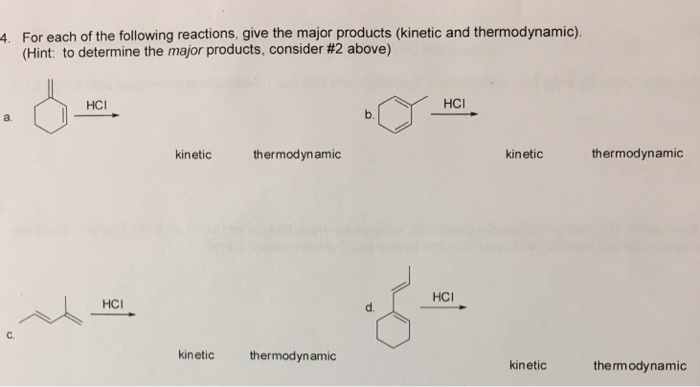For each of the following reactions, give the major products (kinetic and thermodynamic). (Hint: to determine the major products, consider #2 above) 4. HC HCI a. kinetic thermodynamic kinetic thermodynamic HCI HCI d. C. kinetic thermodynamic kinetic the rm odynamic

• ### Student Instructions: Write an essay of at least 2 pages long no more than 4 pages,...

Student Instructions: Write an essay of at least 2 pages long no more than 4 pages, in font Times New Roman, font size 12, and 2 spacing, explaining the following topics about a Balance Scorecard. Submit your essay as an attached WORD document. 1.How a Balanced Scorecard improves financial performance on business. 2.How a Balanced Scorecard aligns business strategy 3.How a Balanced Scorecard convert an organization's values into a defined metric. 4.How a Balance Scorecard is related to the stakeholders.

• ### Consider the reaction Using the standard thermodynamic data in the tables linked above, calculate the equilibrium...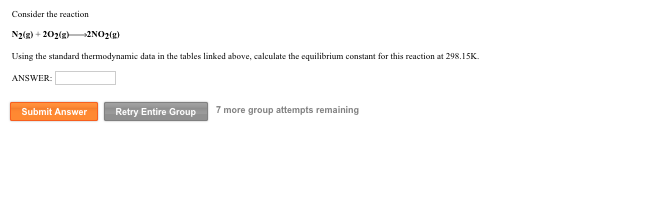Consider the reaction Using the standard thermodynamic data in the tables linked above, calculate the equilibrium constant for this reaction at 298.15K ANSWER Submit Answer Retry Entire Group 7 more group attempts remaining